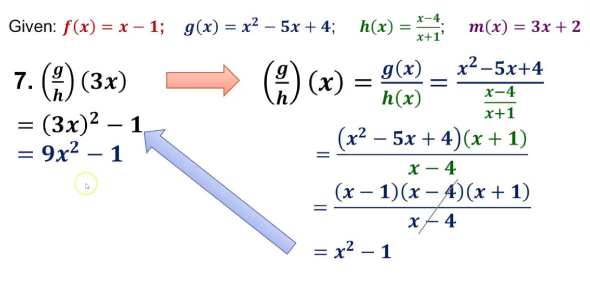# Ultimate General Mathematics Trivia

19 Questions | Total Attempts: 287SettingsHow much do you really know about Mathematics? Find out by taking this quiz!

Related Topics
• 1.
How much water can a cube shaped tank of side 6 cm contain?
• A.

226 cm^3

• B.

56 cm^3

• C.

36 cm^3

• D.

216 cm^3

• 2.
What is the total surface area of a box whose edges are all 12 cm long?
• A.

964 cm^2

• B.

864 cm^2

• C.

144 cm^2

• D.

884 cm^2

• 3.
What is the area of a kite whose diagonals are of 25 and 20 cm?
• A.

120 cm^2

• B.

45 cm^2

• C.

500 cm^2

• D.

250 cm^2

• 4.
If 1.5x = 0.04y, then what is the value of (y - x)≈ (y + x)
• A.

73/77

• B.

Apr-71

• C.

67/73

• D.

112/55

• 5.
How many perfect squares are there in between 120 and 300?
• A.

5

• B.

11

• C.

7

• D.

6

• 6.
Successive discounts of 10, 20, and 30% are similar to a single discount of?
• A.

20%

• B.

49.60%

• C.

60%

• D.

77%

• 7.
If a clock based on oscillating pendulum is taken to moon, how'll it behave?
• A.

Won't change

• B.

It'll stop

• C.

Become slow

• D.

Become fast

• 8.
What is the average of odd numbers up to 100?
• A.

65.5

• B.

50

• C.

45

• D.

44

• 9.
What is the compounded ratio of 5:14 and 7:15?
• A.

5:07

• B.

1:06

• C.

2:03

• D.

6:01

• 10.
Which of these determines the shrillness of a sound?
• A.

Amplitude

• B.

Frequency

• C.

Loudness

• D.

Speed

• 11.
If the cost price of 36 pens = selling price of 30 pens, what's the gain?
• A.

22%

• B.

15%

• C.

10%

• D.

20%

• 12.
What is two-thirds of half of 369?
• A.

228

• B.

123

• C.

318

• D.

339

• 13.
What is the value of 243^0.16 x 243^0.04?
• A.

243

• B.

3

• C.

27

• D.

9

• 14.
1/4th of a tank holds 135L of water, what part of it is full if it holds 180L?
• A.

3-Feb

• B.

2-Jan

• C.

3-Jan

• D.

4-Mar

• 15.
If m of 400 - 7^2 = 159, what is m?
• A.

67

• B.

52

• C.

51

• D.

55

• 16.
How many boxes can accommodate 288 articles with each box containing 24?
• A.

22

• B.

13

• C.

12

• D.

18

• 17.
If in triangle ABC, angle A is 120 degrees and AB = AC, what is angle B?
• A.

75 degrees

• B.

45 degrees

• C.

60 degrees

• D.

30 degrees

• 18.
If a^2 + b^2 + c^2 - ab - bc - ca = 0, then which of these is true?
• A.

A > b = c

• B.

A = b = 2c

• C.

A = b = c

• D.

A = b < c

• 19.
If the sum of two numbers of ratio 1:3 is 240, what is their difference?
• A.

180

• B.

100

• C.

120

• D.

150